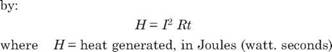## ENERGY SOURCES FOR WELDING

Welding energy sources can be grouped into the following five categories:

— Electrical sources

— Chemical sources

— Optical sources

— Mechanical sources

— Solid state sources.

Of the above sources, electrical sources of energy are more commonly used. Arc and resistance welding will now be highlighted in the following paragraphs.

3.6.1 Arc Welding

• A large number of welding processes use the electric arc as source of heat for fusion. The electric arc consists of a relatively high current discharge sustained through a theramally ionized gaseous column called plasma.

• Power dissipation of the arc is EI (EI cos ф for A. C. welding). Not all of the heat generated in the arc is effectively utilized in the arc welding process. Values of heat utilization may vary from 20 to 85 percent. Efficiency of heat utilization is usually low for GTAW, intermediate for SMAW and high for SAW.

• With higher travel speeds the efficiency of heat transfer in the fusion zone is increased. Thus for the same arc energy input, the volume of fused metal increases as travel speed is increased.• The resistance welding process employ a combination of force and heat to produce a weld between the workpieces. The heat generated by the current flow may be expressed
 ...(3.8)

3.6.2 Resistance Welding

Example. Two sheets of steel 1.0 mm thick are to be spot welded. In ordinary spot welding machine a current of 10,000 A is required for 0.1 second, while with a capacitor dis­charge power source making a projection weld between the same sheets, the current pulse of 30,000 A was required for 0.005 seconds. Compare the two processes. Assume effective resist­ance of 100 ц Q (micro-ohm).

(a) H = (10,000)2 (0.0001) (0.1) = 1000 J (for ordinary spot welding machine)

(b) H = (30,000)2 (0.0001) (0.005) = 450 J (for capacitor discharge power source)

Approx. 1381 J are required to melt 1 g of steel.

Assume that the fusion zone of the above weld is a cylinder of 5 mm diameter and 1 .5 mm height. Weight of metal melted will be (n/4)(5)2 x (1.5) x p = 0.246 g. To heat and melt this mass would require 339 J assuming p = 8.356 x 10-3 g/mm3.

• Thus the capacitor discharge power source utilises energy more effectively.

Комментарии закрыты.